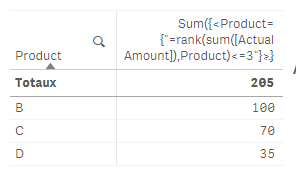# New to Qlik Sense

Discussion board where members can get started with Qlik Sense.

Announcements
Save \$300 - Register for QlikWorld (formerly Qonnections) by January 31st: Learn More
Contributor II

## Multiple Dimension aggregation Columns

Hello Everyone,

From the below sample data table. I want to create an aggregational table and later I will convert it to the scatter plot which shows aggregation of the Products based on their sales amount and Actual amounts. I considered using the ranking for this approach but I am not getting the results as expected.

sample data table:

 Product Sales Amount Actual Amount Risk Value Return Value A 60 5 20 15 B 50 100 25 25 C 70 70 55 35 D 20 35 15 30 E 10 25 17 15 F 5 30 20 20

Expected Result:

 Dimension Risk Value Return Value Average of TOP 3 Products by Sales Amount 33.33 25 Average of TOP 3 Products by Actual Amount 31.66 30

Summary:

Average of TOP 3 Products by Sales Amounts: C,A,B are the Top 3 Products. Now I want Avg(Risk Value) and Avg(Return Value) of these Products.

Average of TOP 3 Products by Actual Amounts: B, C, D are the Top 3 Products. Now I want Avg(Risk Value) and Avg(Return Value) of these Products.

Expressions I am using:

Dimension Expression:

if(aggr(rank(sum( [Sales Amount])),[Product ID])<= 3,'Average of TOP 3 Products by Sales Amounts',

if(Aggr(rank(-sum( [Actual Amount])),[Product ID])<= 3 ,'Average of TOP 3 Products by Actual Amounts'))

Measured Expression:

Avg(Risk Value)

Avg(Return Value)

-Vidya

1 Solution

Accepted SolutionsPartner

## Re: Multiple Dimension aggregation Columns

So basically:

to have the first 3 products by sales amout:

Sum({<Product={"=rank(sum([Sales Amount]),Product)<=3"}>}[Sales Amount])to have the first 3 products by actual amount:

Sum({<Product={"=rank(sum([Actual Amount]),Product)<=3"}>}[Actual Amount])so :

to have the avg of risk value depends on the top 3 based on sales amount:

Avg({<Product={"=rank(sum([Sales Amount]),Product)<=3"}>}[Risk Value])to have the avg of risk value depends on the top 3 based on actual amount:

Avg({<Product={"=rank(sum([Actual Amount]),Product)<=3"}>}[Risk Value])if u want to have this in a table:

as dimension:

=ValueList('Average of TOP 3 Products by Sales Amounts','Average of TOP 3 Products by Actual Amounts')

as a measure:

if(ValueList('Average of TOP 3 Products by Sales Amounts','Average of TOP 3 Products by Actual Amounts') =

'Average of TOP 3 Products by Sales Amounts', Avg({<Product={"=rank(sum([Sales Amount]),Product)<=3"}>}[Risk Value]),

if(ValueList('Average of TOP 3 Products by Sales Amounts','Average of TOP 3 Products by Actual Amounts') =

'Average of TOP 3 Products by Actual Amounts',Avg({<Product={"=rank(sum([Actual Amount]),Product)<=3"}>}[Risk Value])

))

result:4 RepliesPartner

## Re: Multiple Dimension aggregation Columns

So basically:

to have the first 3 products by sales amout:

Sum({<Product={"=rank(sum([Sales Amount]),Product)<=3"}>}[Sales Amount])to have the first 3 products by actual amount:

Sum({<Product={"=rank(sum([Actual Amount]),Product)<=3"}>}[Actual Amount])so :

to have the avg of risk value depends on the top 3 based on sales amount:

Avg({<Product={"=rank(sum([Sales Amount]),Product)<=3"}>}[Risk Value])to have the avg of risk value depends on the top 3 based on actual amount:

Avg({<Product={"=rank(sum([Actual Amount]),Product)<=3"}>}[Risk Value])if u want to have this in a table:

as dimension:

=ValueList('Average of TOP 3 Products by Sales Amounts','Average of TOP 3 Products by Actual Amounts')

as a measure:

if(ValueList('Average of TOP 3 Products by Sales Amounts','Average of TOP 3 Products by Actual Amounts') =

'Average of TOP 3 Products by Sales Amounts', Avg({<Product={"=rank(sum([Sales Amount]),Product)<=3"}>}[Risk Value]),

if(ValueList('Average of TOP 3 Products by Sales Amounts','Average of TOP 3 Products by Actual Amounts') =

'Average of TOP 3 Products by Actual Amounts',Avg({<Product={"=rank(sum([Actual Amount]),Product)<=3"}>}[Risk Value])

))

result:Contributor II

## Re: Multiple Dimension aggregation Columns

Hi Omar,

You are really a genius. I really appreciate your help today. The expression you shared worked as expected. One last thing i would like to share is. I also want to include my own products example: If(wildmatch([Product],'*Micro*'),[Product]). Is there a way that I can add this if condition to the valuelist?

Thanks,

-VidyaPartner

## Re: Multiple Dimension aggregation Columns

If I fully understand ur request:

Let's say you have 1000 products, but only 50 that contains Micro, and u want to to do the Avg for the top 3 of these 50?

Is that so?

If yes, u change ur expression from:

Avg({<Product={"=rank(sum([Sales Amount]),Product)<=3"}>}[Risk Value])

to

Avg({<Product={"=rank(sum(  {<Product={=wildmatch([Product],'*Micro*')=1}>}  [Sales Amount]),Product)<=3"}>}[Risk Value])

so: the final expressions would be:

as dimension:

=ValueList('Average of TOP 3 Products by Sales Amounts','Average of TOP 3 Products by Actual Amounts')

as a measure:

if(ValueList('Average of TOP 3 Products by Sales Amounts','Average of TOP 3 Products by Actual Amounts') =

'Average of TOP 3 Products by Sales Amounts',

Avg({<Product={"=rank(sum({<Product={=wildmatch([Product],'*Micro*')=1}>} [Sales Amount]),Product)<=3"}>}[Risk Value]),

if(ValueList('Average of TOP 3 Products by Sales Amounts','Average of TOP 3 Products by Actual Amounts') =

'Average of TOP 3 Products by Actual Amounts',

Avg({<Product={"=rank(sum({<Product={=wildmatch([Product],'*Micro*')=1}>} [Actual Amount]),Product)<=3"}>}[Risk Value])

))

Contributor II

## Re: Multiple Dimension aggregation Columns

Hi Omar,

I found the solution for this. let's follow the order.

• I have 1000 Products, But only 50 that contains Micro. Now I want to show Avg risk value of top 3 by sales amount of 1000 Products and individual Riks values of products that contains Micro.

Example:Micro1                                                                                                                    10.20

Mirco2                                                                                                                    12.36

• Solution to achieve this requirement is.

Dim

1

2

3

4

5

6

7

8

9

10

];

Dimension:

=pick(Dim,'Average of TOP N Products by AUM: 1 YR Risk and Returns','Average of TOP N Products by 1 YR Flows: 1 YR Risk and Returns','Average of Bottom N Products by 1 YR Flows: 1 YR Risk and Returns',

if(WildMatch([Firm Name],'*Mirco*'),[Product Name]))

Measure:

=

PICK(Dim, Avg({<[Product ID]={"=rank(sum([Sales Amount]),[Product ID])<=10"}>}[Risk Value]),

Avg({<[Product ID]={"=rank(sum([Total Amount]),[Product ID])<=10"}>}[Risk Value]),

Avg({<[Product ID]={"=rank(-sum([Total Amount]),[Product ID])<=10"}>}[Risk Value]),

([Risk Value])

)

Thanks,

-Vidya